Hits: 83

Weibull Distribution

Definition 1: The Weibull distribution has the probability density function (pdf)for x ≥ 0. Here β > 0 is the shape parameter and α > 0 is the scale parameter.

The cumulative distribution function (cdf) isThe inverse cumulative distribution function is I(p) =Observation: There is also a three-parameter version of the Weibull distribution.

Observation: If x represents “time-to-failure”, the Weibull distribution is characterized by the fact that the failure rate is proportional to a power of time, namely β – 1. Thus β can be interpreted as follows:

• A value of β < 1 indicates that the failure rate decreases over time. This happens if there is significant “infant mortality”, or where defective items fail early with a failure rate decreasing over time as the defective items are weeded out of the population.
• A value of β = 1 indicates that the failure rate is constant over time. This might suggest random external events are causing mortality or failure.
• A value of β > 1 indicates that the failure rate increases with time. This happens if there is an “aging” process; e.g. if parts are more likely to wear out and/or fail as time goes on.

1/α can be viewed as the failure rate. The mean of the Weibull distribution is the mean time to failure (MTTF) or mean time between failures (MTBF) =$alpha Gamma ! left( ! 1+frac{1}{beta} ! right)$.

Key statistical properties of the Weibull distribution are:

• Mean =$alpha Gamma ! left( ! 1+frac{1}{beta} ! right)$
• Median =$alpha ({ln 2})^{1/beta}$
• Mode (when β > 1) =$alpha ! left( ! frac{beta-1}{beta} ! right)^{1/beta}$
• Variance =$alpha^2 Gamma ! left( ! 1+frac{2}{beta} ! right)- mu^2$

Excel Function: Excel provides the following function in support of the Weibull distribution.

WEIBULL.DIST(x, βαcum) where α and β are the parameters in Definition 1 and cum = TRUE or FALSE

WEIBULL.DIST(x, βα, FALSE) = the value of the Weibull pdf f(x) at x

WEIBULL.DIST(x, βα, TRUE) = the value of  the Weibull cumulative distribution function F(x) at x

Versions of Excel prior to Excel 2010 use the WEIBULL function instead of the WEIBULL.DIST function.

Example 1: The time to failure of a very sensitive computer screen follows a Weibull distribution with α = 1,000 hours and β = .6. What is the probability that the screen will last more than 5,000 hours? What is the mean time to failure?

The probability that the screen will last no more than 5,000 hours

= WEIBULL.DIST(5000, .6, 1000, TRUE) = 0.92767.

Thus, the probability that the screen will last more than 5,000 hours = 1 – 0.92767 = 7.2%

MTTF = αΓ(1+1/β) = 1000Γ(1+1/.6) = 1000*EXP(GAMMALN(1 + 1/.6)) = 1,504.575 hours

Example 2: If the mean time to failure for a component which follows a Weibull distribution is 1,000 hours with a standard deviation of 400 hours, what is the probability that the component will last more than 2,000 hours?

Thus

We now solve these equations for α and β. First, we simplify the second equation and then we take the natural log of both sides of both equations to get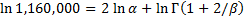Eliminating αwhich is equivalent to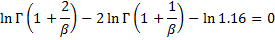The above equation takes the form h(β) = 0, which we solve using Excel’s Goal Seek capability by selecting Data > What If Analysis|Goal Seek and filling in the dialog box that appears as shown in Figure 2.

Once we obtain the value for β, we can calculate α using the equation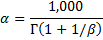and then we can calculate the probability that the component will last more than 2,000 hours using the WEIBULL.DIST function.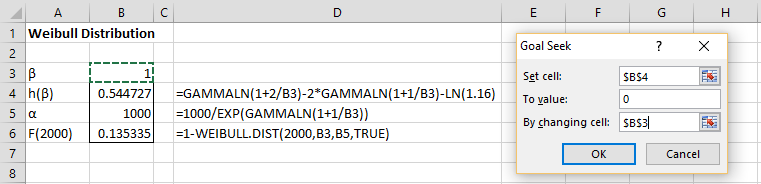Figure 2 – Goal Seek initial guess

After clicking on the OK button, the result is shown in Figure 3.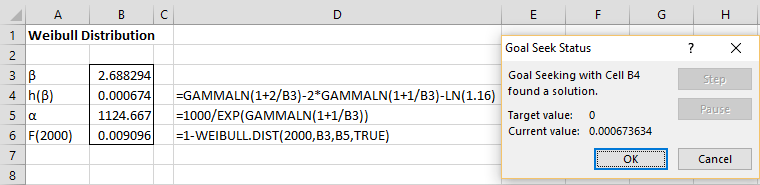Figure 3 – Goal Seek results

The values for α and β are shown in cells B5 and B3. The probability that the component will last more than 2,000 hours is 0.91% (cell B6).

Real Statistics Function: Since Excel doesn’t provide an inverse function, you can use the following function provided by the Real Statistics Resource Pack instead.

WEIBULL_INV(p, βα) = x such that WEIBULL.DIST(x, βα, TRUE) = p; i.e. the inverse of  WEIBULL.DIST(x, βα, TRUE)

Excel formula for Beginners – How to get Sequence of times in Excel

Statistics for Beginners in Excel – Weibull Distribution

Free Machine Learning & Data Science Coding Tutorials in Python & R for Beginners. Subscribe @ Western Australian Center for Applied Machine Learning & Data Science.

Introduction to Applied Machine Learning & Data Science for Beginners, Business Analysts, Students, Researchers and Freelancers with Python & R Codes @ Western Australian Center for Applied Machine Learning & Data Science (WACAMLDS) !!!

Latest end-to-end Learn by Coding Projects (Jupyter Notebooks) in Python and R:

Applied Statistics with R for Beginners and Business Professionals

Data Science and Machine Learning Projects in Python: Tabular Data Analytics

Data Science and Machine Learning Projects in R: Tabular Data Analytics

Python Machine Learning & Data Science Recipes: Learn by Coding

R Machine Learning & Data Science Recipes: Learn by Coding

There are 2000+ End-to-End Python & R Notebooks are available to build Professional Portfolio as a Data Scientist and/or Machine Learning Specialist. All Notebooks are only \$79.95. We would like to request you to have a look at the website for FREE the end-to-end notebooks, and then decide whether you would like to purchase or not.

Please do not waste your valuable time by watching videos, rather use end-to-end (Python and R) recipes from Professional Data Scientists to practice coding, and land the most demandable jobs in the fields of Predictive analytics & AI (Machine Learning and Data Science).

The objective is to guide the developers & analysts to “Learn how to Code” for Applied AI using end-to-end coding solutions, and unlock the world of opportunities!

Disclaimer: The information and code presented within this recipe/tutorial is only for educational and coaching purposes for beginners and developers. Anyone can practice and apply the recipe/tutorial presented here, but the reader is taking full responsibility for his/her actions. The author (content curator) of this recipe (code / program) has made every effort to ensure the accuracy of the information was correct at time of publication. The author (content curator) does not assume and hereby disclaims any liability to any party for any loss, damage, or disruption caused by errors or omissions, whether such errors or omissions result from accident, negligence, or any other cause. The information presented here could also be found in public knowledge domains.